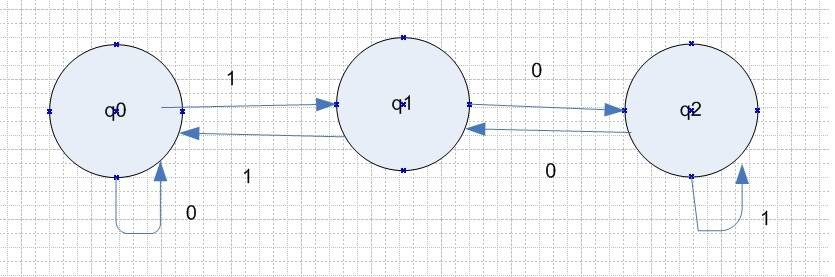# Residue 3 Moore Machine

• Comp Sci
• zak100
zak100
Homework Statement
Create a residue Moore Machine using FA
Relevant Equations
No equations
Hi,
I am trying to create residue 3 automata using Moore. I can't understand why we go to state q1 when we have input 0 in q0 and similalry why we go to q2 when we have input zero in q1.

I have attached an image of FA.

Zulfi.Homework Helper
Gold Member
To analyze this, make sure to start simple.

By definition, the initial state of the state machine is q0. Let's start there.

So what if your input string is the simplest possible input string, "0". One can't get much simpler than that. So now ask yourself, what is 0 mod 3? That should tell you something about how q0 must transition when the input is 0.

Now let's change the input to the next most simple string, "1". What is 1 mod 3? This should tell you something about the transition from q0 when the input is a 1.

Continuing on, what if the input string was "10 (binary; i.e., '2' decimal.)" Ask yourself, "well, what is 2 mod 3?" Do you see now why the state must transition from q1 to q2 when the input is a 0?

Continue with the following strings:
11 (binary): What is 3 mod 3?
100 (binary): What is 4 mod 3?
101 (binary): What is 5 mod 3?

Homework Helper
Gold Member
I can't understand why we go to state q1 when we have input 0 in q0

And for clarity, that's not right. When in state q0, with input 0, the state machine stays in state q0.